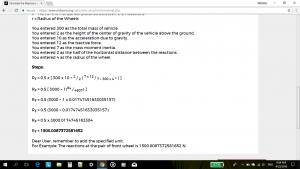## The Calculator For Mechanical Engineers

Nickzom Calculator+ is a 100% all in one package that solves over 32,000 calculations and shows the steps of the calculations. One of the sections it handles is Mechanical Engineering.
Nickzom Calculator+ solves over 5,000+ calculations in Mechanical Engineering and shows the steps, formulas, parameters and answer accurately and comprehensively.

Nickzom Calculator+ handles the following major chapters of Mechanical Engineering:

– Transmission of Power by Belts and Ropes
– Motion of Connected Bodies
– Helical Springs and Pendulum
– Centrifugal Generator | Watt Generator
– Work, Energy and Power
– Motion of Vehicles
– Transmission of Power by Gear Train
– Thermodynamics
– Fluid Mechanics
– Collision of Elastic Bodies
– Motion of Circular Path
– Kinetics of Motion of Rotation
– Hydrostatics
– Principles and Application of Friction
– Principles of Lifting Machines
– Simple Lifting Machines
– Internal Combustion Engine
– Mechanics

Within these major topics there are sub topics right up to selections of the various parameters you desire to find.

To obtain this calculator via web

Visit https://www.nickzom.org/calculator
Scroll to the Bottom
Click on Access Our Professional Version
Register with Us
Activate your Account by Verifying your Email Address
Make a Payment of NGN 1,500
Select Engineering in the Drop Down
Click on “Open Section”
Click on “Mechanical

To obtain this calculator via Google Play Store (For Android)
Visit – https://play.google.com/store/apps/details?id=org.nickzom.nickzomcalculator

To obtain this calculator via Apple App Store (for iOS)
Visit – https://itunes.apple.com/us/app/nickzom-calculator/id1331162702?mt=8

In any case, you search for a particular problem to solve on Mechanical Engineering of which you did not find on this web app. Please, contact us and let us know so that we can implement it immediately for you.

Thank You!##Arithmetic Progression

A sequence is a set of numbers arranged in a definite pattern. Each number is called a term.

A finite sequence is one that has a last term when listed. For Example: 2,4,6,8,,…,16. An infinite sequence is one that does not have a last term when listed.

Arithmetic Progression follows the rule of linear sequence which is the sequence in which each term is obtained by adding a distant number (Positive or Negative) to the proceeding terms.

The constant number is called common difference and it is denoted as “d” while the first term is denoted as “a”.

If T1, T2, T3, T4, T5, … is a linear sequence, the common difference is obtained as:

# What is Simple Interest?

Simple interest is a method of calculating the interest charge on a loan or bill. Simple interest is determined by multiplying the interest rate by the principal by time which is normally in years (per annual) but could be in months, days, weeks etc during the specified time frame.

Simple Interest (S.I.) = (P x r x t) / 100

Where P stands for Principal, r stands for interest rate and t stands for time.

## How to Solve and Apply Simple Interest.

There are a number of different reasons that could make one want to carry out a simple interest operation. One of them being that you want to lend money to your friend to start a business and you want your money to work for you and reproduce during the course of the loan.